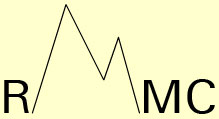Journal of
Integral
Equations
and
Applications

Editorial Board

Information for Authors

Abstracts
and
Complete text

DOI 1988-2007

Subscription Information

Forthcoming Abstracts
and
Articles

RMMC# Journal of Integral Equationsand Applications

Editor

Johannes Tausch
Department of Mathematics
Southern Methodist University
Dallas, Texas 75275-0156 , USA
tausch@mail.smu.edu
(Boundary element methods, fast methods, computational electromagnetics, wavelets)

Associate Editors

Kendall Atkinson
Department of Mathematics
University of Iowa
Iowa City, IA 52242, USA
(Boundary integral equations, the radiosity equation, numerical methods)
,
Yanzhao Cao
Department of Mathematics and Statistics
Auburn University
Auburn, AL 36849, USA
(Theory and numerical methods for Volterra integral equations
and Fredholm integral equations, stochastic integral equations)

Penny Davies
Department of Mathematics
University of Strathclyde
Glasgow, G1 1XH, United Kingdom
(Boundary integral equations, scattering, numerical methods)

Kai Diethelm
Faculty of Applied Natural Sciences and Humanities
University of Applied Sciences W³rzburg-Schweinfurt
97421 Schweinfurt, Germany
(Fractional differential and integral equations, numerical analysis, approximation)

Neville Ford
Department of Mathematics
University of Chester
Chester CH1 4BJ, UK
(Volterra integral and functional equations,
fractional integrals and derivatives, numerical methods)

Paul Martin
Department of Applied Mathematics and Statistics
Golden, CO 80401-1887, USA
(Applications of integral equations, scattering, hypersingular equations)

William McLean
School of Mathematics
University of New South Wales
Sydney, NSW 2052, Australia
(Numerical methods for fractional differential equations, boundary integral equations)

Bernd Silbermann
Technische Universitat Chemnitz
Fakultat fur Mathematik
D-09107 Chemnitz, Germany bernd.silbermann@mathematik.tu-chemnitz.de
(Convolution equations, approximation methods, asymptotic spectral theory)

Ilya Spitkovsky
New York University Abu Dhabi
P.O. Box 129188
Abu Dhabi, United Arab Emirates
(Singular integral equations, convolution equations, boundary value problems for analytic functions)

Da Xu
Department of Mathematics
Hunan Normal University
Changsha 410081, Hunan, People's Republic of China
daxu@hunnu.edu.cn
(Evolutionary integral equation, numerical analysis)

Yuesheng Xu
Department of Mathematics and Statistics
Old Dominion University
Norfolk, VA 23529
Sun Yat-sen University
Guangzhou, China
(Approximation Theory, Boundary Integral Equations, Wavelet Analysis)